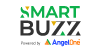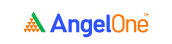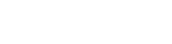# What You Should Know About Glossary of 20 Numbers and Ratios

4.120 numbers and ratios to know

### 1. EBITDA

EBITDA stands for earnings before interest, taxes, depreciation, and amortisation.

 EBITDA = net income + interest + taxes + depreciation + amortisation

### 2. Profit before tax (PBT)

PBT is used to determine a company’s profit before the payment of corporate income tax.

 PBT = revenue - all expenses (except income tax).

### 3. Profit after tax (PAT)

Profit after tax is the final profit that’s available after the company pays off all its expenses and taxes.

 PAT = PBT - taxes

### 4. Profit margin

Profit margin is the percentage of profit a company makes with respect to its revenue.

 Profit margin (%) = (PAT ÷ total sales) x 100

### 5. Earnings per share (EPS)

Earnings per share is the revenue of a company expressed in terms of a single share held in the company.

 EPS = (PAT - dividends for preference shareholders) ÷ total number of equity shares outstanding

### 6. Price to earnings (P/E) ratio

The P/E ratio shows the price that you pay for every 1 rupee of revenue generated by the company.

 P/E ratio = current market price per share ÷ earnings per share

### 7. Dividend per share (DPS)

Dividend per share (DPS) is a metric that’s used to determine the amount of dividend you’re likely to get for every single share you hold in a company.

 DPS = Total amount of dividends paid out in a year (including interim dividends) ÷ number of outstanding equity shares

### 8. Dividend yield

The dividend yield is expressed as a percentage and is used as an indicator of the return on your investment.

 Dividend yield (%) = (dividend per share ÷ price per share) x 100

### 9. Interest coverage ratio

This ratio essentially shows you how much revenue is generated for every rupee of debt interest payment made by a company.

 Interest coverage ratio = EBIT ÷ finance costs

### 10. Current ratio

The current ratio is a useful indicator that’s used to determine a company’s ability to meet its current (short-term) liabilities with its current (short-term) assets.

 Current ratio = current assets ÷ current liabilities

### 11. Quick ratio

Also known as the acid test ratio, quick ratio is an indicator that determines a company’s ability to pay its short-term debts.

 Quick ratio = (current assets - inventory) ÷ current liabilities

### 12. Return on assets (ROA)

Return on assets is a ratio that shows the amount of profit earned by a company for every rupee invested in its assets. It is used to determine the effectiveness of the assets of a company when it comes to generating revenue.

 Return on assets = PAT ÷ average total assets

PAT is basically the net income.

### 13. Return on equity (ROE)

Return on equity shows you just how much of a profit a company generates for every rupee of equity invested in it.

 Return on equity (ROE) in % = (PAT ÷ shareholders’ equity) x 100

### 14. Return on capital employed (ROCE)

Return on capital employed is used to determine how much revenue a company generates with respect to the amount of capital employed by it.

 Return on capital employed (ROCE) in % = [EBIT ÷ overall capital employed] x 100

where,

EBIT = earnings before interest and taxes, and

Overall capital employed = total assets - current liabilities

### 15. Asset turnover ratio

The asset turnover ratio shows the amount of revenue earned by a company for every rupee invested in its assets.

 Asset turnover ratio = revenue ÷ total assets

### 16. Inventory turnover ratio

The inventory turnover ratio shows just how quickly a company is able to sell its inventory (stockpile of produced goods).

 Inventory turnover ratio = cost of goods sold ÷ average inventory

### 17. Debt-equity ratio

This is widely used to determine the ratio of borrowed funds (debt) to that of funds contributed by the shareholders (equity) of a company.

 Debt-equity ratio = total liabilities ÷ total equity

### 18. Debt to asset ratio

This ratio determines the proportion of debt to that of the assets of a company.

 Debt to asset ratio % = (total liabilities ÷ total assets) x 100

### 19. Operating cash flow ratio

Operating cash flow ratio, generally calculated for a specific time period, helps determine the ability of a company to meet all its current (short-term) debts with the cash generated by the company through its operations.

 Operating cash flow ratio = operating cash flow ÷ current liabilities

### 20. Average collection period

This ratio is used to determine the time period a company takes to collect payments owed by its debtors. Average collection period is always calculated for a specific period of time.

 Average collection period = 365 ÷ accounts receivable turnover

Where,

Accounts receivable turnover = revenue from sales ÷ average accounts receivables

How would you rate this chapter?

Get Information Mindfulness!

Catch-up With Market

News in 60 Seconds.The perfect starter to begin and stay tuned with your learning journey anytime and anywhere.